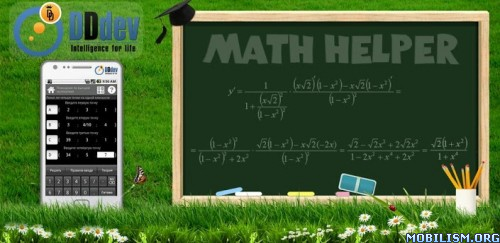# App Releases • Math Helper v3.1.1

Math Helper v3.1.1

Requirements: 2.1 and up

Overview: Universal application of higher mathematics, which helps you not only to solve the problem, but also to see and understand the process of solving it, step by step.A COMPREHENSIVE AND UNIQUE CALCULATOR!

MathHelper’s marks of quality and excellence:

– The application is included in the Intel ® Learning Series Alliance software packs

– It is rated above 4.9 on Google Play with many positive reviews.

Here are just a few user reviews:

> "Perfect companion for the challenges on the linear and probability theories!"

> "Thanks to the authors, for a nominal fee I bought the app without thinking, it will probably cost more for the test for mathematics"

> "Great app. I bought it and don’t regret it. "

This application is a universal assistant for those who deal with advanced mathematics. You can be a higher school student, a university student or even a graduate – If you need immediate mathematical assistance – MathHelper is always at hand!

You can forget about all the heavy books and numerous tricks with the tips, which in most cases do not guarantee that you’ll solve your maths problem.

MathHelper enables you to not only to solve the problem, but also to understand the process of solving it, step by step. This convenient multifunctional calculator includes an extensive theoretical guide in several languages. You’ll get an algebraic mini-laboratory that is stored conveniently and compactly on your Android!

Now, you can not only quickly find the answer, but also handle similar problems in the future. Your classmates and teachers will be very surprised with the results of your work.

MathHelper is indispensable during your math exams! And even if you have already grown out of school uniform and student’s jeans, you can always use a universal mathematical reference book calculator!

MathHelper allows calculating propositions and solving problems in linear and vector algebra in six sections at the moment:

1. Operations with matrices:

* Transpose of a Matrix

* Finding the determinant of a Matrix

* Finding the inverse of a Matrix

* Addition and subtraction of matrices

* Matrix multiplication

* Scalar multiplication of Matrices

* Calculating the rank of a matrix

2. Solving systems of linear equations:

* The Gauss method

* The Cramer’s method

* The Inverse Matrix method

3. Vectors:

* Finding the length (magnitude) of a vector

* Checking if two vectors are collinear

* Orthogonal vectors

* Sum, difference, scalar product

* Finding the cross product

* Finding the angle between two vectors

* Finding the cosine of the angle between two vectors

* Finding the projection of a vector onto another

* Coplanar vectors

4. Figures:

* Finding the area of a triangle

* Show that four points lie on the same plane

* Finding the volume of a tetrahedron (pyramid)

* Finding the volume and height of the tetrahedron (pyramid)

NEW! 5. Mathematical analysis - Derivatives:

* Derivative of the function

* Derivative of the function given parametrically

* The derivative of the implicit function

6. Probability theory:

* Finding expectation sample

* Finding the sampling variance

* Finding the number of permutations of a set of n elements

* Finding the number of placements and combinations (n, k)

7. Number theory and sequence:

* Prime factorization

* Finding GCD and LCM

* The least common multiple and greatest common divisor

* Complex numbers: Addition, subtraction, multiplication and division

* Raising a complex number to a power

* The first terms of a progression (arithmetric and geometric)

* Finding Fibonacci numbers

* Solution of linear Diophantine equations

* Finding the values of Euler’s function

* The factorial n!

Learn math in a new way with MathHelper!

What's New

3.1.0:

- Fixed 16 bugs

- Integration added (requires internet connection and still in beta )

Code:

http://ift.tt/14RfquZ

http://ift.tt/1pTWJzj

Mirror:

http://ift.tt/1pTWHrc

http://ift.tt/NMuinZ

http://ift.tt/NMukfo ... 1.apk.html

DYSholo — Mon Mar 03, 2014 12:08 pm